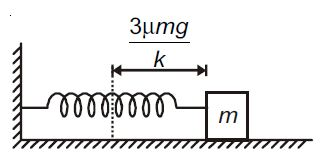# Maximum velocity of the block!A spring of spring constant $k$ connected to a block of mass $m$ is placed on a rough horizontal surface having coefficient of friction $\mu$. The spring is given initial elongation $\frac{3 \mu mg}{k}$ and the block is released from rest. For the subsequent motion, the maximum speed of the block is?

×